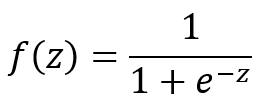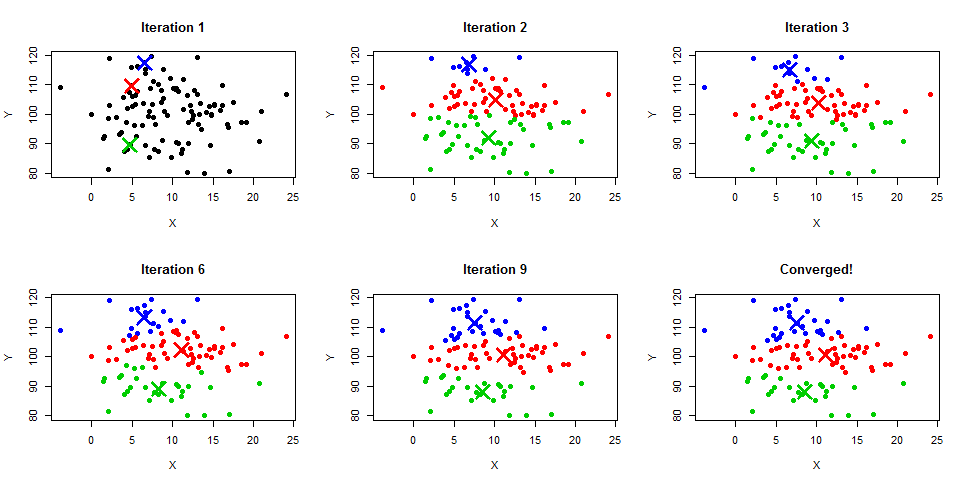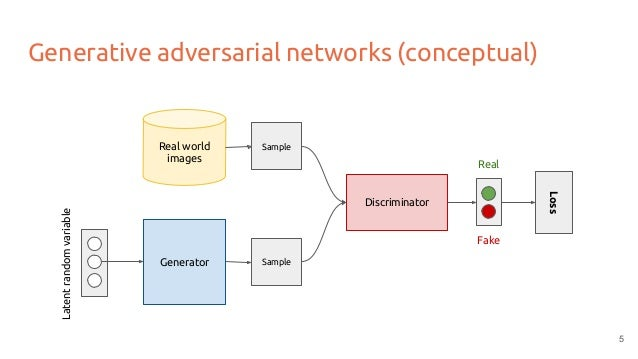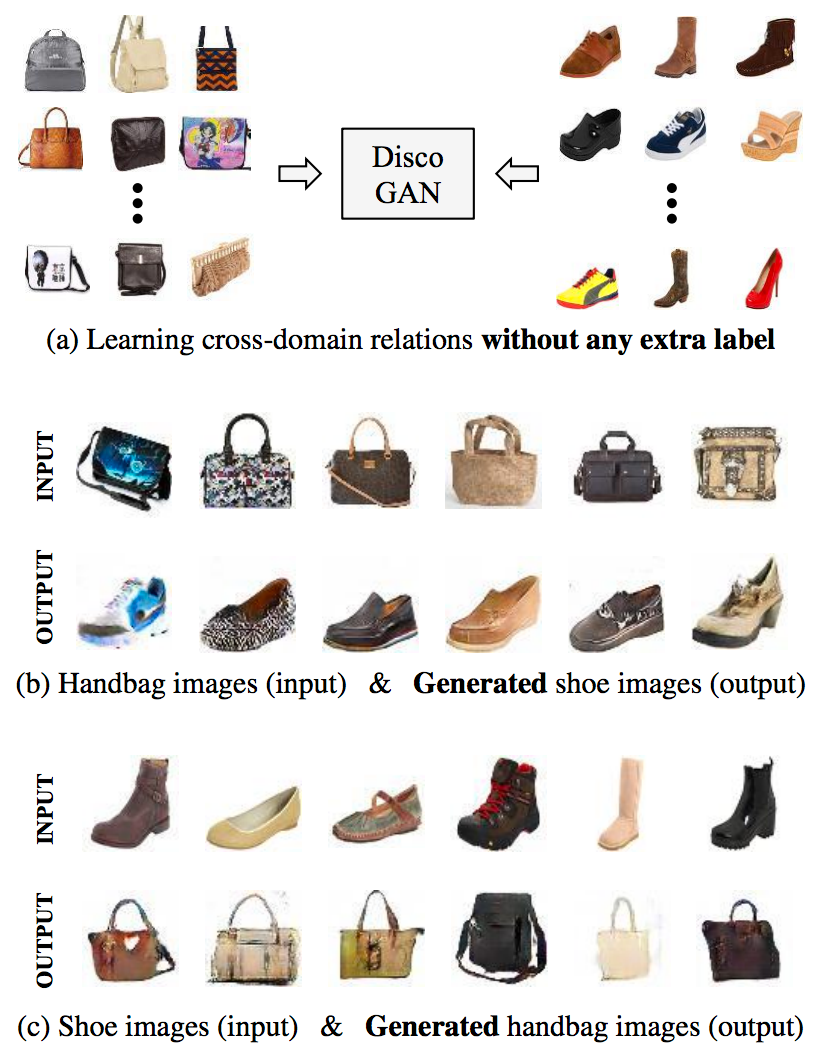## A Brief Overview of Machine Learning

This blog post is going to be a compilation of most common machine learning concepts for those who are getting started on machine learning.

First of all, the relationship between the AI, ML, and deep learning:In one sentence: machine learning is an approach to achieve artificial intelligence, and deep learning is a subfield of machine learning.

# Machine Learning

According to Arthur Samuel in 1959, machine learning gives “computers the ability to learn without being explicitly programmed”. Machine learning tasks are typically divided into:

• Supervised learning: data is labelled — “I tell you: this is a cat and that is a dog. Now guess what this picture is?”
• regression: predict continuous valued output (linear regression: `y = ax + b`)
• classification: predict discrete valued output (models: KNN, mixture of Gaussians, logistic regression, threshold perceptron, logistic perception, etc)
• Unsupervised learning: data is unlabelled — “I don’t know what those images are, but these look like one creature and those images look like another one”
• clustering (e.g., k-means)
• anomaly detection
• Reinforcement learning: machine gets feedback while learning — just like how animals get trained

An algorithm is what is used to train a modelA model is something to which when you give an input, gives an output. Common ML models include:

– Decision tree– Logistic Regression– Neural network– Bayesian network– Support vector machine– Nearest neighbor– k-means– Markov#### Model Comparisons

KNN takes no time to train – memory-intensive (must compute the distances and sort all the training data at each prediction)
– performance depends on the number of dimensions (curse of dimensionality)
– test time efficiency low
Deep NNs – compactly represent a significantly larger set of functions
– eliminates the need for feature engineering
– great for unstructured datasets such as images, audio, and video
– require a long time to train
– computationally expensive
Bayesian networks useful when data is scarce (e.g., medical diagnosis) – computationally expensive (could be NP-hard)
– dependent on quality of prior beliefs
SVM – regularization parameter (avoids overfitting)
– uses kernel trick (defines the similarity function in terms of original space without even knowing what the transformation function K is)
high algorithmic complexity and extensive memory requirements of the required quadratic programming in large-scale tasks (Horváth in Suykens et al. p 392)
Logistic Regression Naive Bayes
estimates the probability(y|x) from training data by minimizing error estimates a joint probability from the training data
splits feature space linearly; doesn’t matter if some features are correlated in case some features are correlated, the prediction might be poor because Naive Bayes assumes conditionally independent features
generally needs a large training dataset in order to draw a good linear separator a small training dataset is fine
Logistic Regression Decision Tree
assumes there is one smooth linear decision boundary assumes that our decision boundaries are parallel to the axes (partitions the feature space into rectangles)

#### How To choose a classifier based on training set size?

First thing we need to be clear about: how do we know if a classifier is better than others? We calculate the validation error for these classifiers and try to find the one with minimum validation error. Now, what is the validation error and how do we find that?

In a typical machine learning application, we split data into 3 sets: 70% training set, 20% cross validation set, and 10% test set. The training set is what’s used for training the model. The cross validation set is what’s used to estimate how well the model has been trained to select the best performing model. The test set is finally used to estimate the accuracy of the selected model. While selecting a model, it’s useful to compare the cross validation error with the training error.

In linear regression, we have the cost functions as follows:

```Jtrain(θ) = 1/2m * ∑(hθ(x(i)) - y(i))2 JCV(θ) = 1/2mcv * ∑(hθ(xcv(i)) - ycv(i))2 Jtest(θ) = 1/2mtest * ∑(hθ(xtest(i)) - ytest(i))2 where θ's are the parameters to the linear function that we define (hθ(x) = θ0 + θ1x + θ2x2 + ...), m is the size of dataset ```

The goal here is to find parameters θ that will minimize the cost functions (errors). As we have a more complex model (i.e., a higher degree of polynomial), we get a graph like this:What is the ideal fit? Low bias and low variance (in the middle of the graph). Unfortunately, it’s almost impossible in practice. Therefore, a bias-variance tradeoff must be made.

See this blog post for an illustration of bias-variance tradeoffs.

Bias: the ability of your model function to approximate the data.

1) Using a linear regression model to model a quadratic relationship will cause a high bias (underfit) because you’ll not be able to approximate the relationship well regardless how you tune your parameters

2) KNN has low bias because it doesn’t assume anything about the distribution of data

High bias (underfit): validation error ≈ training error

-> getting more training data will not help much

-> if training set is small, high bias models tend to perform better because they are less likely to overfit (e.g., Naive Bayes)

Variance: the stability of your model in response to new training example.

1) KNN has high variance (overfit) because it can easily change its prediction if only a few points in the training dataset are changed

2) Linear algorithms tend to have low variance because they have rigid underlying structure that will not change much in the face of new data

High variance (overfit): validation error » training error

-> getting more training data is likely to help (gap can become smaller)

-> if training set is big, high variance models tend to perform better because they can reflect more complex relationships (e.g., logistic regression)

#### Differences between model parameters and model hyperparameters

Model parameters: properties of the training data that are learned by the model on its own. They differ for each experiment.

E.g., weight coefficients (or slope) of a linear regression line and its bias (or y-axis intercept) term, the weights of convolution filters

Model hyperparameters: tuning parameters of an algorithm. They are not to be learned by the model and are set beforehand. They are common for similar models. Running an algorithm over a training dataset with different hyperparameter settings will result in different models.

E.g.,​ regularization penalty, number of hidden layers, learning rate, dropout and gradient clipping threshold, a value for setting the maximum depth of a decision tree

## Neural networks

A neural network is a computing system that are inspired by human brains.

No hidden layer: if your data is linearly separable, then you don’t need any hidden layer

1 hidden layer: can approximate any function that contains a continuous mapping from one finite space to another—- wait, why do we need an additional layer? Let’s take a look at the XOR classic example:

Suppose we are to build a neural network that will produce the XOR truth table.

a b a XOR b
1 1 0
0 1 1
1 0 1
0 0 0

Can you try to represent it with no hidden layer (threshold perceptron)?

Now, can you try to represent it with one hidden layer?

2 hidden layers: can represent an arbitrary decision boundary to arbitrary accuracy with activation functions*note: One hidden layer is sufficient for the majority of problems

(source: Introduction to Neural Networks for Java, Second Edition)

Activation functions produce a non-linear decision boundary of the weighted inputs.## Deep Learning

Deep learning is the application of neural networks with many hidden layers to learning tasks.

When Should You Use Deep Learning? When you’ve got a large training dataset and a good hardware (GPU).

Take a look at why GPUs are necessary for deep learning here.

### Feed-forward neural network:

An acyclic graph that connects neurons.### Convolutional neural networks (CNN):

– made up of neurons that have learnable weights and biases

– local connectivity in many layers -> deep locally connected network -> reduces number of connectionsImportant feature of CNN: weight sharing.This contrasts with ordinary deep neural networks where weights are set arbitrarily:#### Differences between CNN and a fully-connected neural network:

Convolutional neural network Fully connected neural network
Each neuron is only connected to a few nearby (aka local) neurons in the previous layer Each neuron is connected to all neurons in the previous layer
Same set of weights is used for every neuron Weights can vary in each connection
Cheap Expensive in terms of memory (weights) and computation (connections)

#### Why CNN for Image Recognition and Object Detection?

Because CNNs have filters (“locally shared weight layers”) that mimic the human visual system (consider when you recognize a dog: you look at its eyes, its nose, its shape, its color, etc). Each layer of a CNN can be trained to recognize higher level features than the previous layer.

E.g., 1st layer: recognize only edges, blobs and corners.

2nd layer: combine these edges, blobs and corners to identify higher level shapes.

3rd layer: recognize objects like eyes, mouth etc.

last layer: classify the specific objectThis is a good blog post about CNN that is very intuitive to understand.

### Recurrent Neural Networks (RNN)

Outputs are fed back to the network as inputs, as illustrated below:#### Differences between CNN and RNN

CNN RNN
takes a fixed size input and generates fixed-size outputs takes arbitrary size of input and output
a type of feed-forward NN (acyclic) not a type of feed-forward NN (cyclic)
connectivity pattern between neurons are inspired by human visual system (e.g., I see a yellow waterbird with a broad blunt bill, short legs, webbed feet, and a waddling gait — I think it’s a duck) uses time-series information (e.g., It’s been sunny this entire past week, I think it might be sunny again tomorrow)
ideal for images and videos processing ideal for text and speech analysis

Common architectures of RNN: Long short-term memory (LSTM), Gated Recurrent Unit (GRU)

## Unsupervised Learning

### k-means

K-means is an unsupervised machine learning algorithm that finds clusters of data. It starts with randomly initializing k cluster centroids. It keeps grouping data into clusters by closest distance to each centroid, and then it updates the centroids by calculating the new average of points assigned to cluster k. It repeats this process until the algorithm converges.

Illustration:### Anomaly Detection

Anomaly detection identifies events that are not expected to happen. A typical usage is fraud detection. Given an unlabeled test dataset, the detector assumes that the majority of the data are set to be “normal” and looks for data that seems to fit least to others.A discriminative model is one that discriminates between 2 classes of data (real data and fake data)

A generative model doesn’t know anything about classes of data

• Purpose: generate new data which fits the distribution of the training data.
• Objective: be so good at producing fake data that it will fool the discriminator

The discriminator will be tasked with discriminating between samples from the true data X and the artificial data generated by g.

We train both in an alternating manner. Each of their objective can be expressed as a loss function that we can optimize via gradient descent.

Result: both get better at their objectives in tandem. The generator is able to fool the most sophisticated discriminator. This method ends up with generative neural nets that are incredibly good at producing new data.Can you imagine? These beautiful shoes and handbags are completely generated by GAN on its own!source: “Learning to Discover Cross-Domain Relations with Generative Adversarial Networks” by Kim et al. (2017)

That’s it! This concludes a very brief overview of machine learning. I hope you liked this blog post and found it helpful. Please let me know if you have any questions or suggestions!

Special thanks to Rudi Chen, Bai Li, and Michael Tu for comments and suggestions!